A multi-select question (a.k.a. multiple select question) asks the student to mark ALL of the correct options. Students receive points for both marked correct options AND unmarked incorrect options. This type of question is slightly more difficult to grade because the teacher must give points for unmarked incorrect answers. Also, self-grading quizzes using Google Forms with either Flubaroo or the built-in Quiz feature will not correctly grade these questions (they're graded either 100% correct or wrong). In order to correctly grade these questions, we can use this Google Sheets formula:

`=countif(C2,"*correct*")+countif(C2,"*correct*")+countif(C2,"<>*notcorrect*")+countif(C2,"<>*notcorrect*")`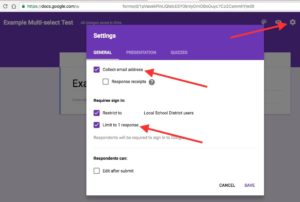Step 1: Create your Google Forms test/quiz as usual. DON'T FORGET to go to settings (the gear icon in the top right) and check Collect Email Address, Restrict To Local School District Users, and Limit To 1 Response.Step 2: Choose Checkboxes from the drop-down box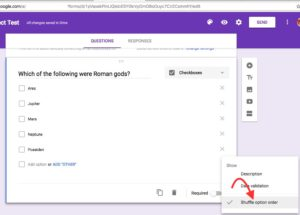Step 3: Shuffle the optionsStep 4: Click the Eye icon and fill out the Answer Key. This can be done before OR after the students take the test/quiz.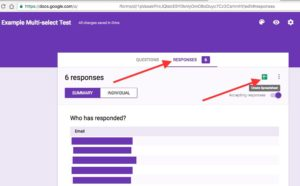Step 5: After students have taken the test/quiz, click Responses and then click the green Google Sheets button. This creates a spreadsheet that contains all of the student responses.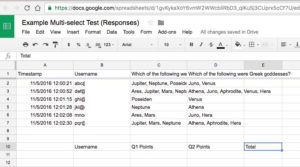Step 6: Beneath all of the student responses, we are going to calculate their scores. You may want to create new headings for this section. For example... Username, Q1 Points, Q2 Points, and Total.Step 7: Use the code
`=B2`
to replicate the first student's username.Step 6b: Copy the first cell and paste it into the cells below it. One way to do this is by clicking the dot in the bottom-right of the cell then dragging down. This will automatically replicate all of the students' usernames.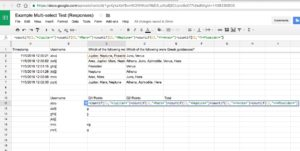Step 7: Copy the Google Sheets formula from the top of the page and paste it in the cell below Q1 points. You will need to change all of the "*correct*" into your correct responses (for example, "*Jupiter*" and "*Mars*" and "*Neptune*" are all correct). You will need to change all of the "<>*incorrect*" into your incorrect responses (for example, "<>*Ares* is an incorrect response and "<>*Poseiden*" is another incorrect). Your finished code will look something like this...
`=countif(C2,"*Jupiter*")+countif(C2,"*Mars*")+countif(C2,"*Neptune*")+countif(C2,"<>*Ares*")+countif(C2,"<>*Poseiden*")`Step 7b: Copy the first Q1 Points cell and paste it into the cells below it. One way to do this is by clicking the dot in the bottom-right of the cell then dragging down. This will automatically calculate all of the students' scores for question 1.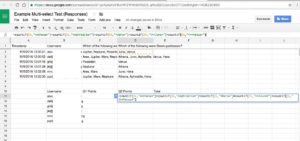Step 7c: Add the
`=countif`
code for other questions. For example, if the correct options were Athena, Aphrodite, and Hera and the incorrect options were Juno and Venus, then your code would look something like this...
`=countif(D2,"*Athena*")+countif(D2,"*Aphrodite*")+countif(D2,"*Hera*")+countif(D2,"<>*Juno*")+countif(D2,"<>*Venus*")`Step 7d: Copy the first Q2 Points cell and paste it into the cells below it. One way to do this is by clicking the dot in the bottom-right of the cell then dragging down. This will automatically calculate all of the students' scores for question 2.Step 8: In the first Total cell, type the code
`=sum(`
and then click-and-drag to select all of the scores for the first student. This will calculate the first student's total score.Step 8b: Copy the first Total cell and paste it into the cells below it. One way to do this is by clicking the dot in the bottom-right of the cell then dragging down. This will automatically calculate all of the students' total scores.

RECENT POSTS